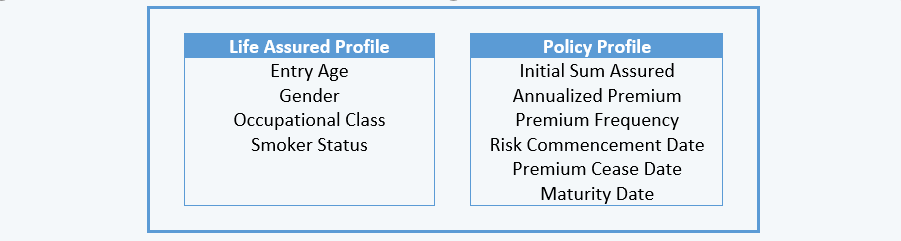# Lesson 3: Calculation A – Movement of Policies

### Understand Model Points

Before setting up formulas used to calculate movement of policies (“MOP”), we need to define the model point used in the calculation, which consist of life assured profile and policy profile: (which are populated in the “input” worksheet)• Model PointModel point is common term in actuarial modeling, which represents a record in an actuarial model to represent a cohort of policies with similar characteristics. A model point may represent ONE policy (seriatim basis) or a group of policies with similar characteristics (grouped basis). For this course, we perform projection on seriatim basis and allow fractional no. of policies (e.g. lx,t = 0.545000).
• Policy term & premium term – Apart from entering premium cease date & maturity date as part of the model point, you may replace the above-mentioned input with policy term & premium term (either in year or month).
• Duration in force in months (“DUR_M”) – DUR_M refers to how long a policy has been set in force, calculated as at valuation date. When we project cash flows for a new business, we shall set DUR_M = 0 (as the policy is not yet set in force). If a policy has DUR_M = 1, it means that as at valuation date (month end), the policy has been in force for 1 month (please note that we assume policies are set in force at the beginning of the month).